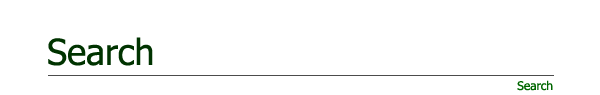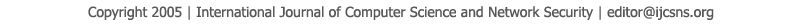To search, Click below search items.All Published Papers Search Service
 Title Author Year Keyword
 Title Accuracy of Data-Model Fit Using Growing Levels of Invariance Models Author Deyab A. Almaleki Citation Vol. 21  No. 12  pp. 157-164 Abstract The aim of this study is to provide empirical evaluation of the accuracy of data-model fit using growing levels of invariance models. Overall model accuracy of factor solutions was evaluated by the examination of the order for testing three levels of measurement invariance (MIV) starting with configural invariance (model 0). Model testing was evaluated by the Chi-square difference test (?χ^2) between two groups, and root mean square error of approximation (RMSEA), comparative fit index (CFI), and Tucker-Lewis index (TLI) were used to evaluate the all-model fits. Factorial invariance result revealed that stability of the models was varying over increasing levels of measurement as a function of variable-to-factor ratio (VTF), subject-to-variable ratio (STV), and their interactions. There were invariant factor loadings and invariant intercepts among the groups indicating that measurement invariance was achieved. For VTF ratio (3:1, 6:1, and 9:1), the models started to show accuracy over levels of measurement when STV ratio was 6:1. Yet, the frequency of stability models over 1000 replications increased (from 69% to 89%) as STV ratio increased. The models showed more accuracy at or above 39:1 STV. Keywords Model- accuracy; Factorial-invariance; Level of measurement invariance; Factorial invariance URL http://paper.ijcsns.org/07_book/202112/20211223.pdf